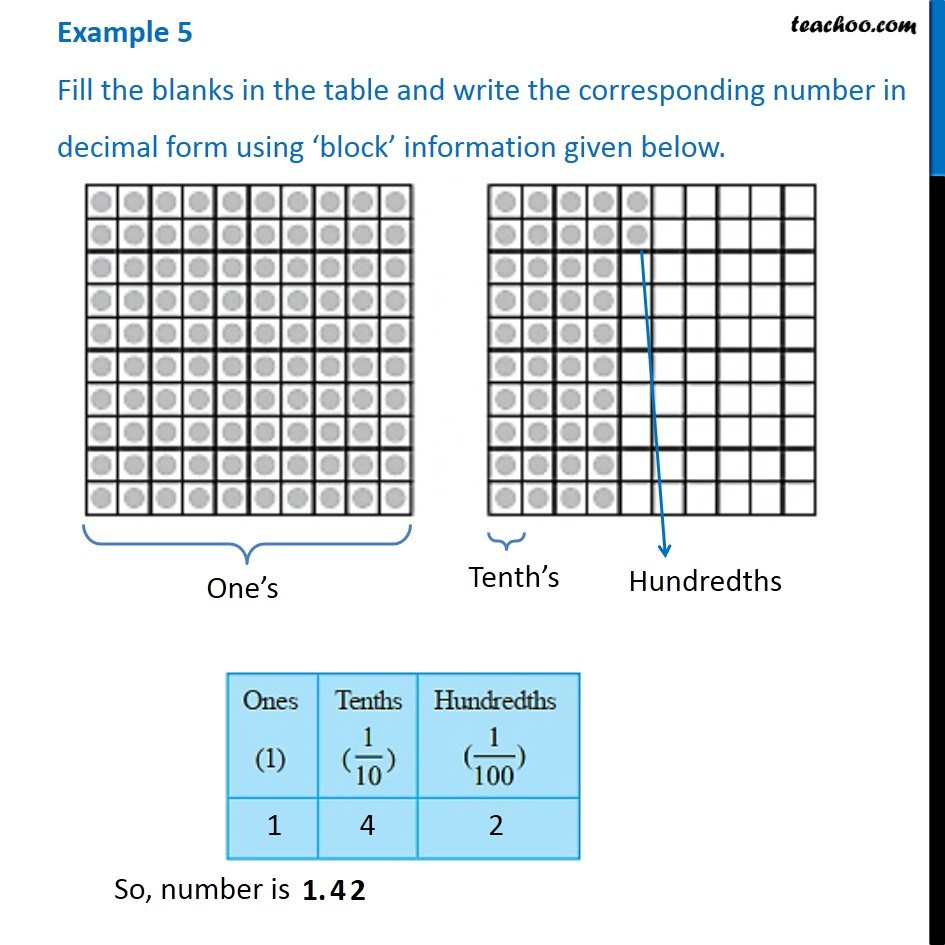1. Chapter 8 Class 6 Decimals
2. Serial order wise
3. Examples

Transcript

Example 5 - Chapter 8 Class 6 Decimals Fill the blanks in the table and write the corresponding number in decimal form using block information given below. Here, 1 dot is Hundredth, 10 dots is Tenth's And 100 dots is One's So, we have 1 - Ones (1) 4 - Tenths (1/10) 2 - Hundredths (1/100) So, number is 1.42